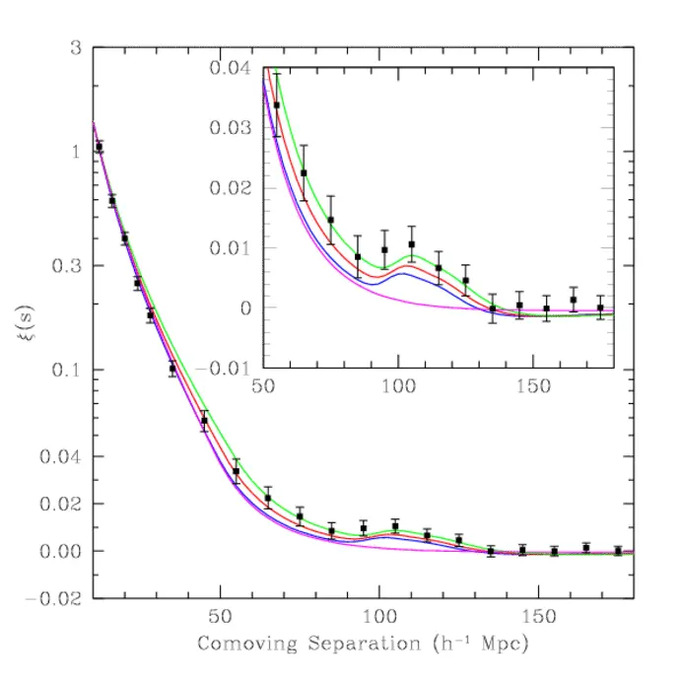Galaxy Clustering

Galaxy clustering refers to the 3D distribution of galaxies, measured from the angular positions of galaxies in the sky, and the redshifts of the galaxies. Galaxy clustering enables the direct measurement of the cosmic expansion history, H(z), through baryon acoustic oscillations (BAO), and the growth history of cosmic large scale structure, fg(z), through redshift-space distortions (RSD) on large scales.

At the last scattering of cosmic microwave background (CMB) photons (an epoch also known as recombination), the acoustic oscillations in the photon-baryon fluid became frozen, and imprinted their signatures on both the CMB (the acoustic peaks in the CMB angular power spectrum) and the matter distribution (BAO in the galaxy power spectrum). Because baryons comprise only a small fraction of matter, and the matter power spectrum has evolved significantly since last scattering of photons, BAO are much smaller in amplitude than the CMB acoustic peaks, and are washed out on small scales by nonlinear growth of matter clustering. BAO in the observed galaxy power spectrum have the characteristic scale, s, determined by the comoving sound horizon at recombination, which is precisely measured by the CMB anisotropy data. Thus BAO can be used as a cosmological standard ruler.The first conclusive detection of baryon acoustic oscillations in the spherically averaged galaxy correlation function, using data of Sloan Digital Sky Survey luminous red galaxies. Image Credit: Eisenstein et al. (2005)

In the observed galaxy distribution, the BAO scale appears as a slightly preferred comoving length scale, corresponding to a slightly preferred redshift separation of galaxies in the radial direction, dz, and a slightly preferred angular separation of galaxies in the transverse direction, dθ. Comparing the observed BAO scales with the expected values gives H(z) in the radial direction [via an application of Hubble's Law: c*dz=H(z)*s], and the angular diameter distasnce DA(z) =r(z)/(1+z) [where r(z) is the comoving distance] in the transverse direction [via the small angle approximation: dθ=s/DA(z)].

We cannot measure the distances to galaxies directly; we can only measure the redshifts of galaxies (which can be converted into distances for a known cosmological model). On very large scales, galaxies fall toward overdense regions under the influence of gravity. This leads to a distortion in the redshift distribution of galaxies [hence the name "redshift-space distortions" (RSD)] on the very large scales, with the degree of distortion proportional to the growth rate of large scale strcuture, fg(z). The measurement of fg(z) can be obtained through independent measurements of the linear RSD parameter β=fg(z)/b, and the bias parameter b(z) (which describes how light traces mass). The parameter β can be measured directly from galaxy redshift survey data by studying the observed redshift-space correlation function of galaxies. The bias parameter can be measured from higher order correlation functions of galaxies, or the weak lensing shear of galaxies. RSD need to be modelled carefully in order to extract information on fg(z), since the random motions of galaxies on small scales also lead to RSD, which are likely coupled to the nonlinear matter clustering effects on those scales.

The systematic effects of BAO as a standard ruler are: the bias between galaxy and matter distributions, nonlinear effects of matter clustering on small and intermediate scales, and redshift-space distortions. Cosmological N-body simulations are required to quantify these effects.

Euclid will carry out a galaxy redshift survey over ~15,000 square degrees using slitless spectroscopy, measuring the redshifts of ~30 million Hα emission line galaxies over the redshft range of 0.9-1.8, complementing ground-based galaxy redshift survey BOSS (which covers the redshift range of 0.1-0.7).